Search by Topic

Resources tagged with Working systematically similar to Shape Draw:

Filter by: Content type:
Age range:
Challenge level:

There are 340 results

Broad Topics > Using, Applying and Reasoning about Mathematics > Working systematically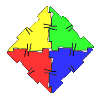Polydron

Age 7 to 11 Challenge Level:

This activity investigates how you might make squares and pentominoes from Polydron.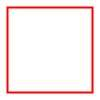Numerically Equal

Age 7 to 11 Challenge Level:

Can you draw a square in which the perimeter is numerically equal to the area?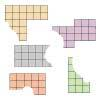Torn Shapes

Age 7 to 11 Challenge Level:

These rectangles have been torn. How many squares did each one have inside it before it was ripped?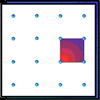Geoboards

Age 7 to 11 Challenge Level:

This practical challenge invites you to investigate the different squares you can make on a square geoboard or pegboard.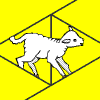Fencing Lambs

Age 7 to 11 Challenge Level:

A thoughtful shepherd used bales of straw to protect the area around his lambs. Explore how you can arrange the bales.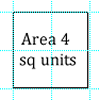Dicey Perimeter, Dicey Area

Age 7 to 11 Challenge Level:

In this game for two players, you throw two dice and find the product. How many shapes can you draw on the grid which have that area or perimeter?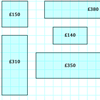Through the Window

Age 7 to 11 Challenge Level:

My local DIY shop calculates the price of its windows according to the area of glass and the length of frame used. Can you work out how they arrived at these prices?More Transformations on a Pegboard

Age 7 to 11 Challenge Level:

Use the interactivity to find all the different right-angled triangles you can make by just moving one corner of the starting triangle.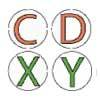Coordinate Challenge

Age 7 to 11 Challenge Level:

Use the clues about the symmetrical properties of these letters to place them on the grid.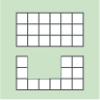Area and Perimeter

Age 7 to 11 Challenge Level:

What can you say about these shapes? This problem challenges you to create shapes with different areas and perimeters.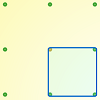Peg and Pin Boards

Age 5 to 11

This article for teachers suggests activities based on pegboards, from pattern generation to finding all possible triangles, for example.Making Squares

Age 7 to 11

Investigate all the different squares you can make on this 5 by 5 grid by making your starting side go from the bottom left hand point. Can you find out the areas of all these squares?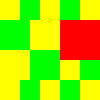Tiles on a Patio

Age 7 to 11 Challenge Level:

How many ways can you find of tiling the square patio, using square tiles of different sizes?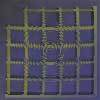My New Patio

Age 7 to 11 Challenge Level:

What is the smallest number of tiles needed to tile this patio? Can you investigate patios of different sizes?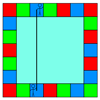Ribbon Squares

Age 7 to 11 Challenge Level:

What is the largest 'ribbon square' you can make? And the smallest? How many different squares can you make altogether?Nine-pin Triangles

Age 7 to 11 Challenge Level:

How many different triangles can you make on a circular pegboard that has nine pegs?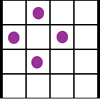Square Corners

Age 7 to 11 Challenge Level:

What is the greatest number of counters you can place on the grid below without four of them lying at the corners of a square?Tiling

Age 7 to 11 Challenge Level:

An investigation that gives you the opportunity to make and justify predictions.Making Boxes

Age 7 to 11 Challenge Level:

Cut differently-sized square corners from a square piece of paper to make boxes without lids. Do they all have the same volume?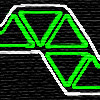Tri-five

Age 7 to 11 Challenge Level:

Find all the different shapes that can be made by joining five equilateral triangles edge to edge.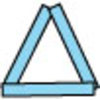Sticks and Triangles

Age 7 to 11 Challenge Level:

Using different numbers of sticks, how many different triangles are you able to make? Can you make any rules about the numbers of sticks that make the most triangles?Triangles All Around

Age 7 to 11 Challenge Level:

Can you find all the different triangles on these peg boards, and find their angles?Uncanny Triangles

Age 7 to 11 Challenge Level:

Can you help the children find the two triangles which have the lengths of two sides numerically equal to their areas?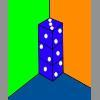Dice in a Corner

Age 7 to 11 Challenge Level:

How could you arrange at least two dice in a stack so that the total of the visible spots is 18?Shapes on the Playground

Age 7 to 11 Challenge Level:

Sally and Ben were drawing shapes in chalk on the school playground. Can you work out what shapes each of them drew using the clues?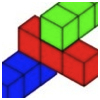Building with Longer Rods

Age 7 to 14 Challenge Level:

A challenging activity focusing on finding all possible ways of stacking rods.Age 11 to 14 Challenge Level:

How many different symmetrical shapes can you make by shading triangles or squares?Hexpentas

Age 5 to 11 Challenge Level:

How many different ways can you find of fitting five hexagons together? How will you know you have found all the ways?Putting Two and Two Together

Age 7 to 11 Challenge Level:

In how many ways can you fit two of these yellow triangles together? Can you predict the number of ways two blue triangles can be fitted together?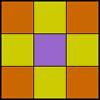Symmetry Challenge

Age 7 to 11 Challenge Level:

Systematically explore the range of symmetric designs that can be created by shading parts of the motif below. Use normal square lattice paper to record your results.Tri.'s

Age 7 to 11 Challenge Level:

How many triangles can you make on the 3 by 3 pegboard?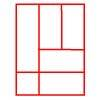Is a Square a Rectangle?

Age 7 to 11 Challenge Level:

How many rectangles can you find in this shape? Which ones are differently sized and which are 'similar'?Egyptian Rope

Age 7 to 11 Challenge Level:

The ancient Egyptians were said to make right-angled triangles using a rope with twelve equal sections divided by knots. What other triangles could you make if you had a rope like this?Cover the Tray

Age 7 to 11 Challenge Level:

These practical challenges are all about making a 'tray' and covering it with paper.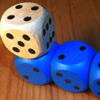The Dice Train

Age 7 to 11 Challenge Level:

This dice train has been made using specific rules. How many different trains can you make?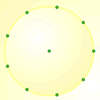Age 7 to 11 Challenge Level:

How many DIFFERENT quadrilaterals can be made by joining the dots on the 8-point circle?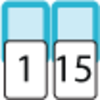Fifteen Cards

Age 7 to 11 Challenge Level:

Can you use the information to find out which cards I have used?Order the Changes

Age 7 to 11 Challenge Level:

Can you order pictures of the development of a frog from frogspawn and of a bean seed growing into a plant?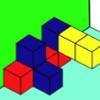Three Sets of Cubes, Two Surfaces

Age 7 to 11 Challenge Level:

How many models can you find which obey these rules?Arranging the Tables

Age 7 to 11 Challenge Level:

There are 44 people coming to a dinner party. There are 15 square tables that seat 4 people. Find a way to seat the 44 people using all 15 tables, with no empty places.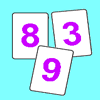Penta Primes

Age 7 to 11 Challenge Level:

Using all ten cards from 0 to 9, rearrange them to make five prime numbers. Can you find any other ways of doing it?Seven Flipped

Age 7 to 11 Challenge Level:

Investigate the smallest number of moves it takes to turn these mats upside-down if you can only turn exactly three at a time.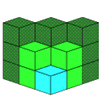Age 7 to 11 Challenge Level:

If you had 36 cubes, what different cuboids could you make?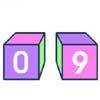Calendar Cubes

Age 7 to 11 Challenge Level:

Make a pair of cubes that can be moved to show all the days of the month from the 1st to the 31st.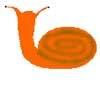Snails' Trails

Age 7 to 11 Challenge Level:

Alice and Brian are snails who live on a wall and can only travel along the cracks. Alice wants to go to see Brian. How far is the shortest route along the cracks? Is there more than one way to go?Six Is the Sum

Age 7 to 11 Challenge Level:

What do the digits in the number fifteen add up to? How many other numbers have digits with the same total but no zeros?The Pet Graph

Age 7 to 11 Challenge Level:

Tim's class collected data about all their pets. Can you put the animal names under each column in the block graph using the information?Roll These Dice

Age 7 to 11 Challenge Level:

Roll two red dice and a green dice. Add the two numbers on the red dice and take away the number on the green. What are all the different possible answers?Teddy Town

Age 5 to 14 Challenge Level:

There are nine teddies in Teddy Town - three red, three blue and three yellow. There are also nine houses, three of each colour. Can you put them on the map of Teddy Town according to the rules?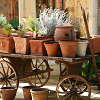Seven Pots of Plants

Age 7 to 11 Challenge Level:

There are seven pots of plants in a greenhouse. They have lost their labels. Perhaps you can help re-label them.